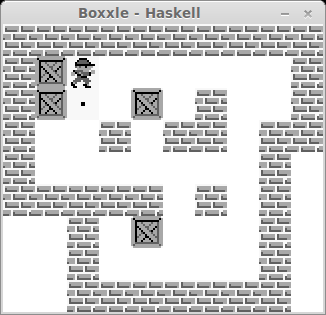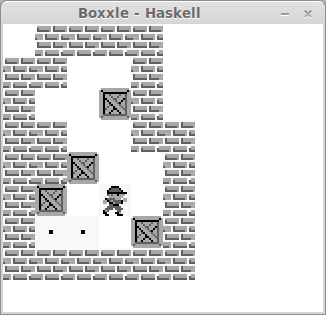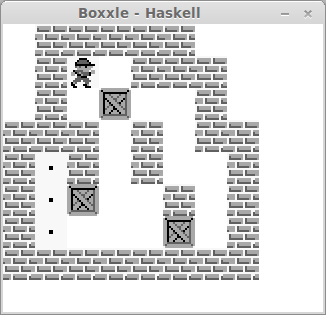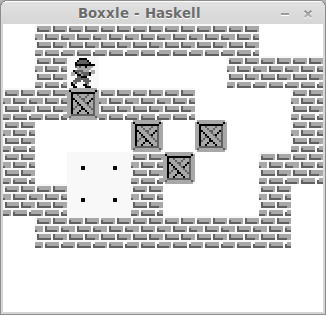## Boxxle in Haskell + SDL

The main driver for me studying programming in the first place was because I love programming video games. After the discovery of SDL bindings for Haskell, along with an amazing tutorial, I couldn't resist trying to make a simple game in Haskell. It took a lottttttt of reading and time in GHCI before I could start making any real progress, but after a couple days I began to wrap my head around things. This is a small remake of the GameBoy game Boxxle, a very simple but fun puzzle game.

The full code (with 40 levels) can be found on my GitHubBelow is the current version. I am still wrapping my head around the intricacies of Haskell, so feel free to make suggestions :)

Boxxle.hs

{-# LANGUAGE FlexibleContexts #-} -- http://stackoverflow.com/questions/10865963/using-the-state-monad-to-hide-explicit-state

-- @author Kenny Cason
-- kennycason.com 2013

import Graphics.UI.SDL
import Graphics.UI.SDL.TTF as TTFG
import Graphics.UI.SDL.Mixer

import Data.List

import Timer

-- game data
-- levels from: http://www.gamefaqs.com/gameboy/585643-boxxle/faqs/52416

rooms = [
roomBuilder
[[1,1,1,1,1,0,0,0,0]                    -- map tiles
,[1,0,0,0,1,0,0,0,0]
,[1,0,0,0,1,0,1,1,1]
,[1,0,0,0,1,0,1,4,1]
,[1,1,1,0,1,1,1,4,1]
,[0,1,1,0,0,0,0,4,1]
,[0,1,0,0,0,1,0,0,1]
,[0,1,0,0,0,1,1,1,1]
,[0,1,1,1,1,1,0,0,0]
]
[(Coord 2 2), (Coord 3 2), (Coord 2 3)] -- boxes
[(Coord 7 3), (Coord 7 4), (Coord 7 5)] -- targets
(Coord 1 1)                                -- start pos
,roomBuilder
[[1,1,1,1,1,1,1,1,1,1]
,[1,4,4,0,0,0,0,0,0,1]
,[1,4,4,0,0,0,1,0,0,1]
,[1,0,0,1,0,1,1,0,1,1]
,[1,0,0,0,0,0,0,0,1,0]
,[1,1,1,1,1,0,1,0,1,0]
,[0,0,1,0,0,0,0,0,1,0]
,[0,0,1,0,0,0,0,0,1,0]
,[0,0,1,1,1,1,1,1,1,0]
]
[(Coord 3 2), (Coord 4 3), (Coord 2 4), (Coord 4 6)]
[(Coord 1 1), (Coord 2 1), (Coord 1 2), (Coord 2 2)]
(Coord 1 1)
,roomBuilder
[[0,1,1,1,1,0]
,[1,1,0,0,1,0]
,[1,0,0,0,1,0]
,[1,1,0,0,1,1]
,[1,1,0,0,0,1]
,[1,4,0,0,0,1]
,[1,4,4,0,4,1]
,[1,1,1,1,1,1]
]
[(Coord 2 2), (Coord 2 3), (Coord 3 4), (Coord 2 5)]
[(Coord 1 5), (Coord 1 6), (Coord 2 6), (Coord 4 6)]
(Coord 1 2)
,roomBuilder
[[0,1,1,1,1,1,0,0]
,[0,1,0,0,1,1,1,0]
,[0,1,0,0,0,0,1,0]
,[1,1,1,0,1,0,1,1]
,[1,4,1,0,1,0,0,1]
,[1,4,0,0,0,1,0,1]
,[1,4,0,0,0,0,0,1]
,[1,1,1,1,1,1,1,1]
]
[(Coord 3 2), (Coord 2 5), (Coord 5 6)]
[(Coord 1 4), (Coord 1 5), (Coord 1 6)]
(Coord 2 1)
,roomBuilder
[[0,1,1,1,1,1,1,1,0,0]
,[0,1,0,0,0,0,0,1,1,1]
,[1,1,0,1,1,1,0,0,0,1]
,[1,0,0,0,0,0,0,0,0,1]
,[1,0,4,4,1,0,0,0,1,1]
,[1,1,4,4,1,0,0,0,1,0]
,[0,1,1,1,1,1,1,1,1,0]
]
[(Coord 2 2), (Coord 4 3), (Coord 6 3), (Coord 5 4)]
[(Coord 2 4), (Coord 3 4), (Coord 2 5), (Coord 3 5)]
(Coord 2 1)
]

roomBuilder ::[[Int]] -> [Coord] -> [Coord] -> Coord -> Room
roomBuilder tiles boxes targets startPos = Room {
tiles = tiles
,walls = foldTiles tiles
,boxes = boxes
,targets = targets
,startPos = startPos
}

-- global constants
startLevel = 1

tEmpty     = 0
tBrick     = 1
tBox     = 2
tPlayer = 3
tTarget    = 4

textColor = Color 0x33 0x33 0x33

-- type defines
data Direction = UP | DOWN | LEFT | RIGHT deriving (Eq, Enum)

data Move = Move {
dir :: Direction
,dx :: Int
,dy :: Int
}

data Coord = Coord {
x :: Int
,y :: Int
} deriving (Eq)

data Room = Room {
tiles :: [[Int]]
,walls :: [Coord]
,boxes :: [Coord]
,targets :: [Coord]
,startPos :: Coord
}

data GameData = GameData {
timer :: Timer
,room :: Room
,player :: Coord
,level :: Int
}

data GameConfig = GameConfig {
screen :: Surface
,sprites :: Surface
,front :: Font
,music :: Music
}

type GameState = StateT GameData IO
type GameEnv = ReaderT GameConfig GameState

getGameData :: MonadState GameData m => m GameData
getGameData = get

putGameData :: MonadState GameData m => GameData -> m ()
putGameData = put

modifyGameData :: MonadState GameData m => (GameData -> GameData) -> m ()
modifyGameData = modify

getPlayer :: MonadState GameData m => m Coord
getPlayer = gets player

getRoom :: MonadState GameData m => m Room
getRoom = gets room

getLevel :: MonadState GameData m => m Int
getLevel = gets level

getTimer :: MonadState GameData m => m Timer
getTimer = gets timer

putTimer :: MonadState GameData m => Timer -> m ()
putTimer t = modify $\s -> s { timer = t } modifyTimerM :: MonadState GameData m => (Timer -> m Timer) -> m () modifyTimerM act = getTimer >>= act >>= putTimer getScreen :: MonadReader GameConfig m => m Surface getScreen = liftM screen ask getSprites :: MonadReader GameConfig m => m Surface getSprites = liftM sprites ask getFont :: MonadReader GameConfig m => m Font getFont = liftM front ask -- main functions newGame :: Int -> IO (GameConfig, GameData) newGame lvl = do -- setVideoMode 320 288 32 [] setVideoMode 448 352 32 [] setCaption "Boxxle - Haskell" [] screen <- getVideoSurface sprites <- loadBMP "img/boxxle.bmp" font <- openFont "fonts/steelpla.ttf" 18 timer <- start defaultTimer openAudio 22050 AudioS16Sys 2 4096 music <- loadMUS "music/main.wav" playMusic music (-1) return (GameConfig screen sprites font music, GameData timer room (startPos room) lvl) where room = (rooms !! (lvl - 1)) levelUp :: GameData -> GameData levelUp gd = gd { level = newLevel, room = nextRoom, player = (startPos nextRoom) } where newLevel = (level gd) + 1 nextRoom = rooms !! ((level gd) mod (length rooms)) resetLevel :: GameData -> GameData resetLevel gd = gd { room = resetRoom, player = (startPos resetRoom) } where resetRoom = rooms !! ( ((level gd) - 1) mod (length rooms) ) handleWin :: GameData -> GameData handleWin gd| isWin = levelUp gd | otherwise = gd where isWin = length (intersect (boxes currentRoom) (targets currentRoom)) == length (targets currentRoom) where currentRoom = (room gd) foldTiles :: [[Int]] -> [Coord] foldTiles tiles = [Coord x y -- generate a Coord pair | (y, row) <- enumerate tiles -- for each row with its coordinate , (x, tile) <- enumerate row -- for each tile in the row (with coordinate) , tile == 1] -- if the tile is 1 where enumerate = zip [0..] getSpriteSheetOffset :: Int -> Maybe Rect getSpriteSheetOffset n = Just (Rect offx offy 32 32) where offx = n * 32 offy = 0 applySurface :: Int -> Int -> Surface -> Surface -> Maybe Rect -> IO Bool applySurface x y src dst clip = blitSurface src clip dst offset where offset = Just Rect { rectX = x, rectY = y, rectW = 0, rectH = 0 } drawSprite :: Surface -> Surface -> Int -> Int -> Int -> IO Bool drawSprite screen sprites n x y = blitSurface sprites (getSpriteSheetOffset n) screen dst where dst = Just (Rect x y 32 32) drawPlayer :: Surface -> Surface -> Int -> Int -> IO Bool drawPlayer screen sprites x y = blitSurface sprites src screen dst where src = (getSpriteSheetOffset tPlayer) dst = Just (Rect (x * 32) (y * 32) 32 32) drawTile :: Surface -> Surface -> Int -> [Coord] -> IO() drawTile screen sprites tile coords = mapM_ (\c -> drawSprite screen sprites tile ((x c) * 32) ((y c) * 32) ) coords drawBricks :: Surface -> Surface -> [Coord] -> IO() drawBricks screen sprites bricks = drawTile screen sprites tBrick bricks drawBoxes :: Surface -> Surface -> [Coord] -> IO() drawBoxes screen sprites boxes = drawTile screen sprites tBox boxes drawTargets :: Surface -> Surface -> [Coord] -> IO() drawTargets screen sprites targets = drawTile screen sprites tTarget targets collide :: Coord -> Coord -> Bool collide c1 c2 = ((x c1) == (x c2)) && ((y c1) == (y c2)) offsetCoord :: Coord -> Move -> Coord offsetCoord c move = c { x = (x c) + (dx move), y = (y c) + (dy move) } collideWithWorld :: Coord -> Room -> Bool collideWithWorld c room = (foldr (||) False (map (collide c) (walls room))) collideWithBoxes :: Coord -> Room -> Bool collideWithBoxes c room = (foldr (||) False (map (collide c) (boxes room))) canBoxMove :: Coord -> Room -> Bool canBoxMove box room = not ((collideWithWorld box room) || (collideWithBoxes box room)) movePlayer :: Move -> GameData -> GameData movePlayer move gd | collideWithWorld newPlayerPos (room gd) = gd | otherwise = gd { player = Coord { x = (x playerPos) + (dx move), y = (y playerPos) + (dy move)} } where playerPos = (player gd) newPlayerPos = (offsetCoord playerPos move) moveBox :: Move -> Coord -> Coord moveBox move box = box { x = (x box) + (dx move), y = (y box) + (dy move) } checkBox :: Room -> Coord -> Move -> Coord -> Coord checkBox room playerPos move box| collidedWithPlayer && (dir move) == UP && boxCanMove = moveBox move box | collidedWithPlayer && (dir move) == DOWN && boxCanMove = moveBox move box | collidedWithPlayer && (dir move) == LEFT && boxCanMove = moveBox move box | collidedWithPlayer && (dir move) == RIGHT && boxCanMove = moveBox move box | otherwise = box where collidedWithPlayer = collide playerPos box -- player collided boxCanMove = canBoxMove newBoxPos room where newBoxPos = offsetCoord box move checkBoxes :: Room -> Coord -> Move -> [Coord] -> [Coord] checkBoxes room playerPos move boxes = map (checkBox room playerPos move) boxes handleBoxes :: Move -> GameData -> GameData handleBoxes move gd = gd { room = (room gd) { boxes = (checkBoxes (room gd) playerPos move (boxes (room gd)) ) } } where playerPos = offsetCoord (player gd) move -- if the resulting move yields the player standing on a box, undo it undoPlayer :: Move -> GameData -> GameData undoPlayer move gd | collideWithBoxes playerPos (room gd) = gd { player = Coord { x = (x playerPos) - (dx move), y = (y playerPos) - (dy move)} } | otherwise = gd where playerPos = (player gd) handleKeyboard :: Event -> GameData -> GameData handleKeyboard (KeyDown (Keysym SDLK_UP _ _)) gd = ((handleWin.undoPlayer move).(movePlayer move).(handleBoxes move)) gd where move = Move { dir = UP, dx = 0, dy = -1 } handleKeyboard (KeyDown (Keysym SDLK_DOWN _ _)) gd = ((handleWin.undoPlayer move).(movePlayer move).(handleBoxes move)) gd where move = Move { dir = DOWN, dx = 0, dy = 1 } handleKeyboard (KeyDown (Keysym SDLK_LEFT _ _)) gd = ((handleWin.undoPlayer move).(movePlayer move).(handleBoxes move)) gd where move = Move { dir = LEFT, dx = -1, dy = 0 } handleKeyboard (KeyDown (Keysym SDLK_RIGHT _ _)) gd = ((handleWin.undoPlayer move).(movePlayer move).(handleBoxes move)) gd where move = Move { dir = RIGHT, dx = 1, dy = 0 } handleKeyboard (KeyDown (Keysym SDLK_r _ _)) gd = resetLevel gd handleKeyboard (KeyDown (Keysym SDLK_s _ _)) gd = levelUp gd handleKeyboard _ d = d loop :: GameEnv () loop = do timer <- getTimer screen <- getScreen sprites <- getSprites pos <- getPlayer room <- getRoom font <- getFont level <- getLevel message <- liftIO$ renderTextSolid font ("level " ++ (show level)) textColor

modifyTimerM $liftIO . start quit <- whileEvents$ modifyGameData . handleKeyboard

liftIO $do bgRect <- Just liftM getClipRect screen white <- mapRGB' screen 0xff 0xff 0xff fillRect screen bgRect white drawBricks screen sprites (walls room) drawTargets screen sprites (targets room) drawBoxes screen sprites (boxes room) drawPlayer screen sprites (x pos) (y pos) applySurface 340 3 message screen Nothing Graphics.UI.SDL.flip screen ticks <- getTimerTicks timer when (ticks < secsPerFrame)$ do
delay $secsPerFrame - ticks unless quit loop where framesPerSecond = 30 secsPerFrame = 1000 div framesPerSecond mapRGB' = mapRGB . surfaceGetPixelFormat whileEvents :: MonadIO m => (Event -> m ()) -> m Bool whileEvents act = do event <- liftIO pollEvent case event of Quit -> return True NoEvent -> return False _ -> do act event whileEvents act runLoop :: GameConfig -> GameData -> IO () runLoop = evalStateT . runReaderT loop main = withInit [InitEverything]$ do -- withInit calls quit
result <- TTFG.init
if not result
then putStr "Failed to init ttf\n"
else do
(gc, gd) <- newGame startLevel
runLoop gc gd
haltMusic
closeAudio
TTFG.quit